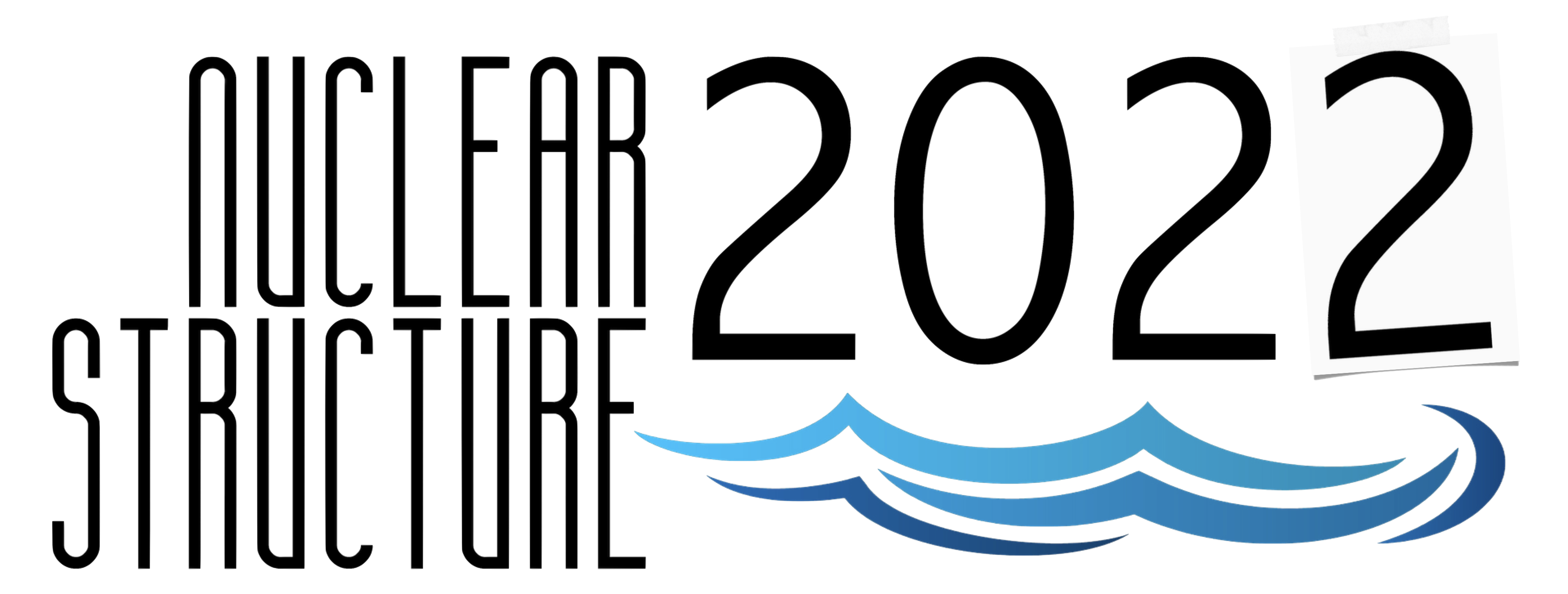#Nuclear Structure 2022

Jun 13 – 17, 2022
Berkeley, CA
US/Pacific timezone

## Extending the Hoyle-state paradigm to $^{12}$C+$^{12}$C fusion

Not scheduled
1m
Berkeley, CA

#### Berkeley, CA

Lawrence Berkeley National Laboratory
Oral Poster Presentations

### Speaker

David Jenkins (University of York)

### Description

Carbon burning is a key step in the evolution of massive stars, Type 1a supernovae and superbursts in x-ray binary systems. Determining the $^{12}$C+$^{12}$C fusion cross section at energies relevant to these different astrophysical scenarios by extrapolation of direct measurements is challenging due to resonances at and below the Coulomb barrier.

A study of the $^{24}$Mg(α,α′)$^{24}$Mg reaction has recently identified several 0$^+$ states in $^{24}$Mg, close to the $^{12}$C+$^{12}$C threshold, which predominantly decay by $^{20}$Ne(g.s)+α. These states were not observed in $^{20}$Ne(α, α$_0$)$^{20}$Ne resonance scattering suggesting that they may have a dominant $^{12}$C+$^{12}$C cluster structure. Given the very low angular momentum associated with sub-barrier fusion, these states may play a decisive role in $^{12}$C+$^{12}$C fusion in analogy to the Hoyle state in helium burning. This demonstrates how nuclear structure is important to various aspects of nuclear astrophysics. Estimates of updated $^{12}$C+$^{12}$C fusion reaction rates are presented based on contributions from these near-threshold 0$^+$ states.

### Primary author

David Jenkins (University of York)

### Presentation materials

There are no materials yet.